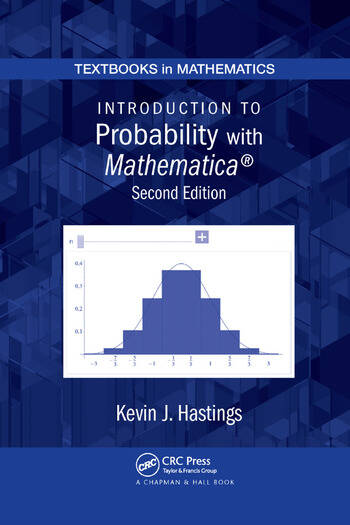Introduction to Probability with Mathematica

2nd Edition

Kevin J. Hastings

Chapman and Hall/CRC
Published September 21, 2009
Reference - 465 Pages
ISBN 9781420079388 - CAT# C7938

For Instructors Request Inspection Copy

USD\$120.00

FREE Standard Shipping!

Preview

Summary

Updated to conform to Mathematica® 7.0, Introduction to Probability with Mathematica®, Second Edition continues to show students how to easily create simulations from templates and solve problems using Mathematica. It provides a real understanding of probabilistic modeling and the analysis of data and encourages the application of these ideas to practical problems. The accompanying CD-ROM offers instructors the option of creating class notes, demonstrations, and projects.

New to the Second Edition

• Expanded section on Markov chains that includes a study of absorbing chains

• New sections on order statistics, transformations of multivariate normal random variables, and Brownian motion

• More example data of the normal distribution

• More attention on conditional expectation, which has become significant in financial mathematics

• Additional problems from Actuarial Exam P

• New appendix that gives a basic introduction to Mathematica

• New examples, exercises, and data sets, particularly on the bivariate normal distribution

• New visualization and animation features from Mathematica 7.0

• Updated Mathematica notebooks on the CD-ROM (Go to Downloads/Updates tab for link to CD files.)

After covering topics in discrete probability, the text presents a fairly standard treatment of common discrete distributions. It then transitions to continuous probability and continuous distributions, including normal, bivariate normal, gamma, and chi-square distributions. The author goes on to examine the history of probability, the laws of large numbers, and the central limit theorem. The final chapter explores stochastic processes and applications, ideal for students in operations research and finance.

Instructors

We provide complimentary e-inspection copies of primary textbooks to instructors considering our books for course adoption.